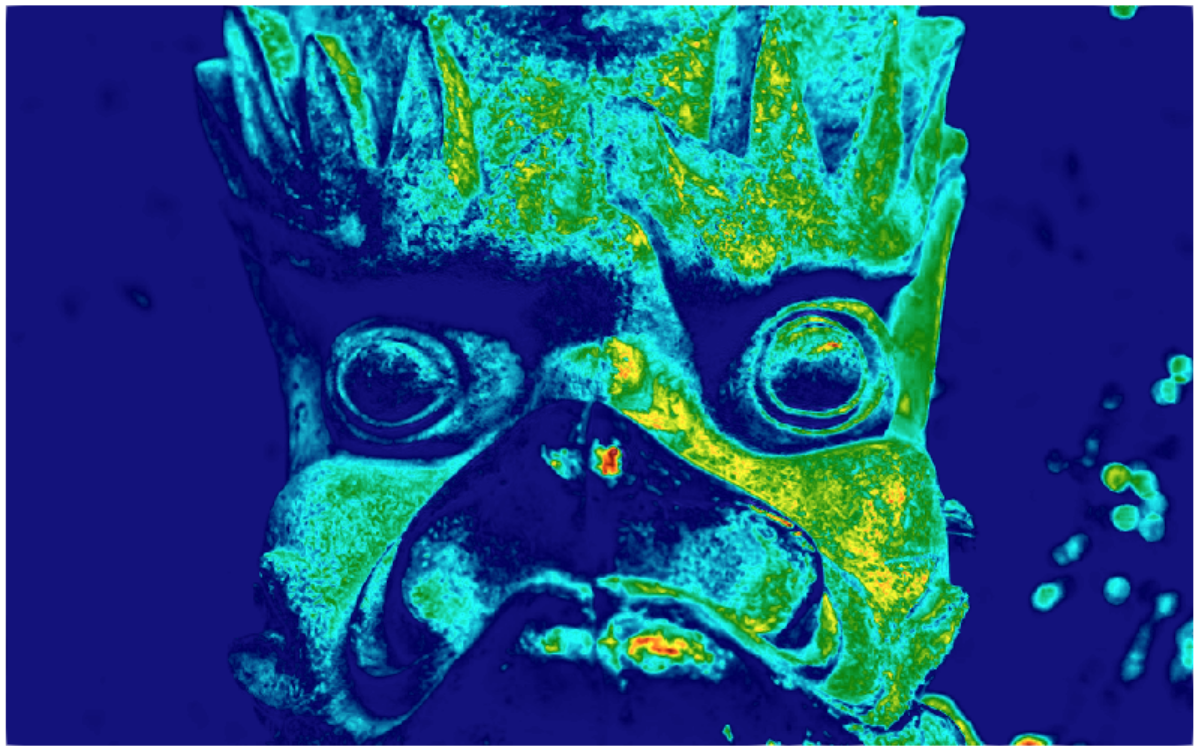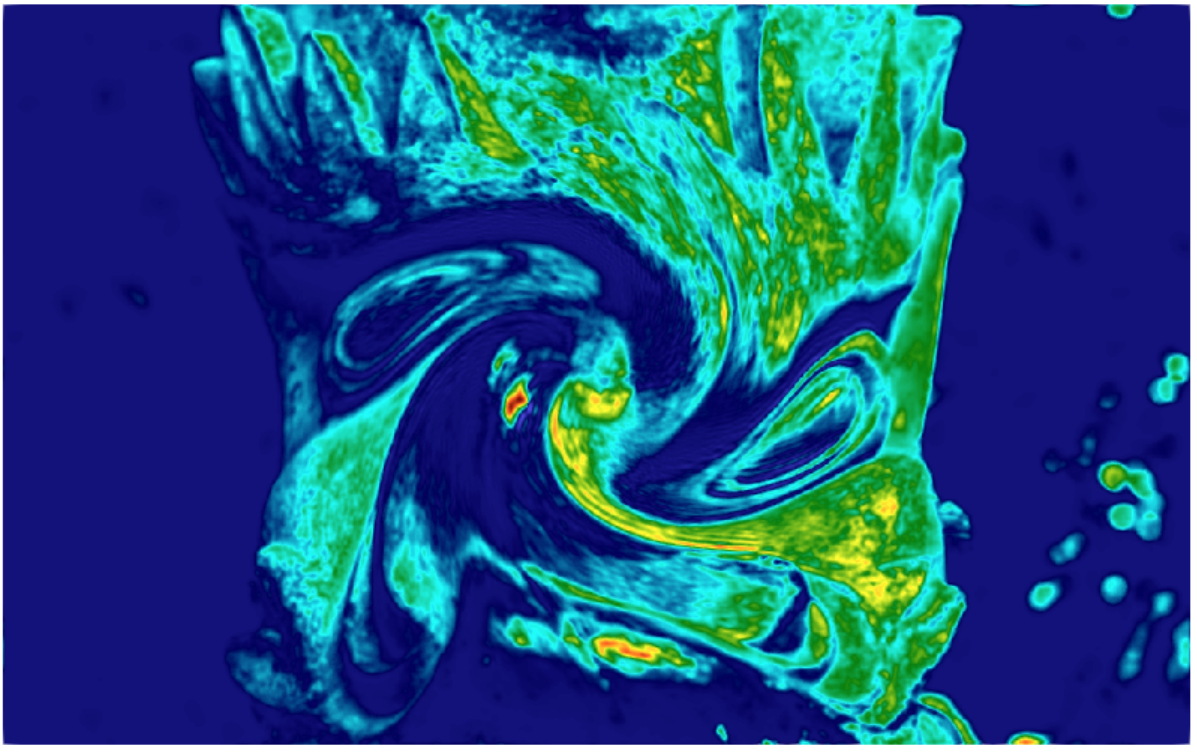# Example of initalization using RunR

This tutorial presents an example of how to use R for initialization of simulation.

## To run it

make configure
./configure --enable-rinside # don't forget to add --other-flags-you-use
tools/install.sh --rpackage 'png'
# alternatively one can install the package from R console: $install.packages("png") make advection_diffusion2D_fields CLB/advection_diffusion2D_fields/main example/runr/advection_diffusion_fields.xml  ## What it does It is a simple example of adding to a model (in this case advection_diffusion2D) the capability to be initialized from "parametric" fields. By parametric field, we mean any field which is accessible only in a said lattice node (doesn't have a stencil). Such parametric fields, can be declared with parameter=TRUE argument to AddField or AddDensity. From the perspective of the code they are easier to operate on, as writing to them doesn't require exchange of information through MPI. Such "parametric" fields can be accessed through RunR, and in turn used for setting custom profiles of velocities, densities, phasefields, etc. The example file executes the following R code: library(png) tab = readPNG("example/data/japan.png")  Reads the png (requires png package) nx = dim(tab) ny = dim(tab) v = as.vector(tab[,,3]^3) x = round(as.vector(Solver$Geometry$X) - 0.5) %% nx y = round(as.vector(Solver$Geometry$Y) - 0.5) %% ny Solver$Fields$phi0[] = v[(ny - 1 - y) + ny*x + 1]  Writes the third (blue) component of the png to the field phi0 (raised to the third power, for contrast). One could do Solver$Fields$phi0[] = tab[,,3], but that would not cope with the orientation and potential MPI division. R = 100 maxu = 0.01 x = Solver$Geometry$X - 512 y = Solver$Geometry$Y - 320 r = sqrt(x^2+y^2) w = exp(-r^2/(2*R^2)) / (exp(-1/2)*R) * maxu Solver$Fields$ux[] = y * w Solver$Fields$uy[] = -x * w  Calculates and sets the advection velocities fields ux and uy with a vortex around middle of the domain. Solver$Actions\$InitFromFields() # Initiate phi from phi0


Executes the InitFromFields action, which in this case just does phi=phi0